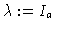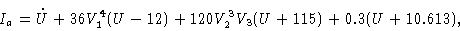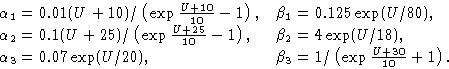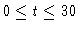## Hodgkin-Huxley Nerve Model

exd14
Variables and parameters:

U (t): membrane potential of a nerve cell

V1, V2,V3: artificial variables defining permeability of the membrane

Ia: applied current (bifurcation parameter)transfer rates:Figure 1
(U,V1)-phase diagram of the Hodgkin-Huxley equation; two trajectories: U(0)=-5 and -6, with V1(0)=0.31, V2(0)=0.05, V3(0)=0.6 and Ia=0.

Figure 2
U(t) for; Hodgkin-Huxley equation; two trajectories: U(0)=-5 and -6, with V1(0)=0.31, V2(0)=0.05, V3(0)=0.6 and Ia=0.

Figure 3
(U,V1)-phase diagram of the Hodgkin-Huxley equation; trajectory approaching a limit cycle. U(0)=-5, V1(0)=0.31, V2(0)=0.05, V3(0)=0.6 and Ia=-10.

Figure 4
(U,V1)-phase diagram of the Hodgkin-Huxley equation; six trajectories U(0)=U0, with V1(0)=0.31, V2(0)=0.05, V3(0)=0.6 and Ia=0; U0=-5.0/-5.2/-5.4/-5.6/-5.8/-6.0

Figure 5
U(t) for; membrane potential of the Hodgkin-Huxley equation; six trajectories U(0)=U0, with V1(0)=0.31, V2(0)=0.05, V3(0)=0.6 and Ia=0; U0=-5.0/-5.2/-5.4/-5.6/-5.8/-6.0

This Example
Figure 1
Figure 2
Figure 3
Figure 4
Figure 5